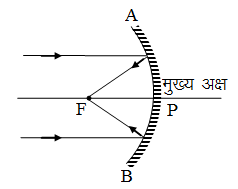# Physics

### Chapter : 3. Light Reflection

#### Reflection From Spherical Mirror

Reflection From Spherical Mirror
INTRODUCTION : There are two types of spherical mirrors:
(i) Concave mirror :(ii) Convex mirror :Some Terms Associated With Spherical Mirrors.
Aperture. The diameter of the circular rim of the mirror. In diagram AB is the aperture of the mirror.
Pole : The centre of the spherical surface of the mirror is called the pole of the mirror. It lies on the surface. In diagram, P is the pole of the mirror.
Centre of curvature : The centre of the spherical shell, of which the mirror is a section, is called centre of curvature of the mirror. It lies outside the surface. Every point on mirror surface lies at same distance from it. In diagram, C is the centre of curvature of the mirror.
Principal axis : The straight line passing through the pole and the centre of curvature of the mirror, is called principal axis of the mirror.
Principal focus : It is a point on the principal axis of the mirror, such that the rays incident on the mirror parallel to the principal axis after reflection, actually meet at this point (in case of a concave mirror) or appear to come from it (in case of a convex mirror). In diagram, F is the principal focus of the mirror.
Radius of curvature : The distance between the pole and the centre of curvature of the mirror, is called the radius of curvature of the mirror. It is equal to the radius of the spherical shell of which the mirror is a section. In diagram, PC is the radius of curvature of the mirror. It is represented by the symbol R.
Focal length : The distance between the pole and principal focus of the mirror, is called the focal length of the mirror. In diagram, PF is the focal length of the mirror. It is represented by the symbol f.
F = + R/2 for Convex Lens
F = – R/2 for Concave Lens
Principal section : A section of the spherical mirror cut by a plane passing through its centre of curvature and the pole of the mirror, is called a principal section of the mirror. It contains the principal axis. In diagram, APB is the principal section of the mirror cut by the plane of the book page.